﻿ 关于我国地区及城乡与消费支出结构的有关分析 Relevant Analysis on the Structure ofConsumption Expenditure in China’s Regions, Urban and Rural Areas

Statistics and Application
Vol. 07  No. 05 ( 2018 ), Article ID: 27203 , 12 pages
10.12677/SA.2018.75061

Relevant Analysis on the Structure of Consumption Expenditure in China’s Regions, Urban and Rural Areas

Yu Liu

College of Sciences, North China University of Technology, Beijing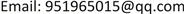Received: Oct. 1st, 2018; accepted: Oct. 15th, 2018; published: Oct. 22nd, 2018ABSTRACT

With the rapid development of China’s economy, the life of residents is also changing, which is reflected in their consumption structure. In order to better understand the current state of China’s economic operation, it is an indispensable link to study the structure of consumer expenditure in various regions. This paper mainly studies the relationship between regions and consumption structure expenditure, disposable income and consumption structure. Specifically, R language program was used to analyze the structure of per capita consumption expenditure in different regions. Next using R language and SAS programs, it made a canonical correlation analysis of urban and rural disposable income and consumption expenditure structure to explore the differences of the consumption expenditure structure between urban and rural areas in our country, and put forward the beneficial suggestion to reduce the difference.

Keywords:Disposable Income, Structure of Consumer Spending, Correspondence Analysis, Canonical Correlation AnalysisCopyright © 2018 by author and Hans Publishers Inc.1. 绪论

2. 我国各地区与消费支出结构对应分析

2.1. 对应分析部分 

${\chi }^{2}$ 值等于4792.5，p值远小于0.05，所以拒绝原假设，认为选取的地区因素与消费结构支出方向不独立，即两种因素之间有密切联系，可进一步进行对应分析。对应分析结果如图1所示。Table 1. 31 consumption structure expenditure table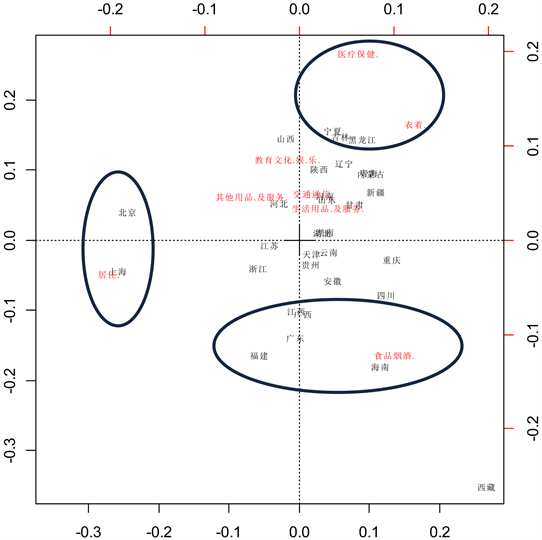Figure 1. 31 area and consumption structure correspondence analysis chart

2.2. 结论部分

3. 我国四大地区与消费支出结构的对应分析

3.1. 对应分析部分

3.2. 结论部分Table 3. Consumption structure expenditure in four major regions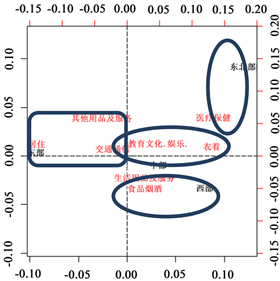Figure 2. Correspondence analysis chart of four regions and consumption structure

4. 城镇居民人均可支配收入与消费结构的典型相关分析

4.1. 典型相关分析部分Table 5. Correlation between income group and consumption structure expenditure groupTable 6. Correlations among the consumption structure expenditure group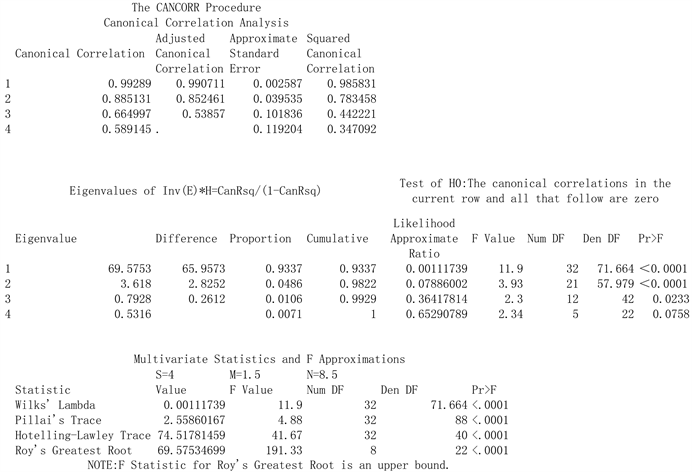Figure 3. Significance test of canonical correlation analysis in cities and towns

[,1] [,2] [,3] [,4]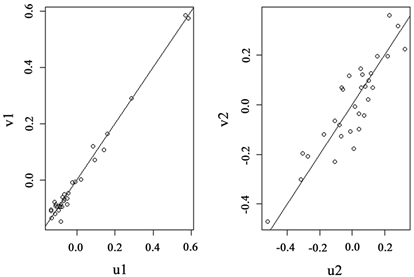Figure 4. Scatter diagram of variables

[,1] [,2] [,3] [,4]

${U}_{1}=0.10616571{X}_{1}+0.00425469{X}_{2}+0.06819492{X}_{3}+0.02254345{X}_{4}$

$\begin{array}{c}{V}_{1}=-0.011890987{Y}_{1}+0.002120268{Y}_{2}+0.153697099{Y}_{3}+0.022450837{Y}_{4}\\ +0.035792618{Y}_{5}+0.010284472{Y}_{6}-0.030751116{Y}_{7}+0.002614506{Y}_{8}\end{array}$

${U}_{2}=0.06044959{X}_{1}-0.03254398{X}_{2}-0.20662300{X}_{3}+0.22435161{X}_{4}$

$\begin{array}{c}{V}_{2}=0.08086345{Y}_{1}+0.05663192{Y}_{2}-0.01557558{Y}_{3}-0.04048317{Y}_{4}\\ -0.20699157{Y}_{5}+0.02753423{Y}_{6}+0.22206740{Y}_{7}-0.03466661{Y}_{8}\end{array}$

${U}_{3}=0.21690198{X}_{1}+0.16039792{X}_{2}-0.27874769{X}_{3}+0.08906621{X}_{4}$

$\begin{array}{c}{V}_{3}=0.13090008{Y}_{1}+0.07792958{Y}_{2}-0.35401080{Y}_{3}-0.05019458{Y}_{4}\\ +0.12013831{Y}_{5}+0.13211165{Y}_{6}+0.02451187{Y}_{7}+0.02872814{Y}_{8}\end{array}$

4.2. 结论部分

5. 农村居民人均可支配收入与消费结构的典型相关分析

5.1. 典型相关分析部分 

[,1] [,2] [,3] [,4]

[,1] [,2] [,3] [,4] [,5]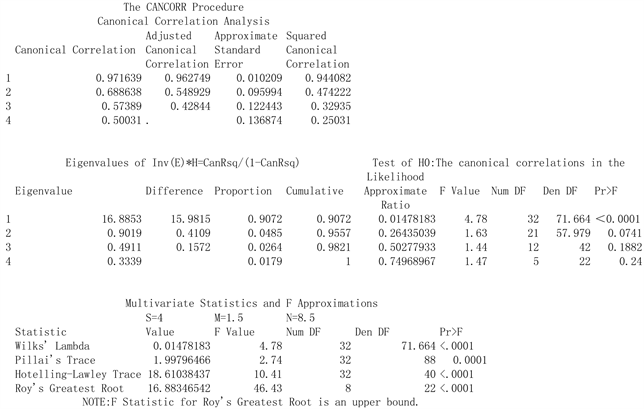Figure 5. Significance test of canonical correlation analysis in rural areas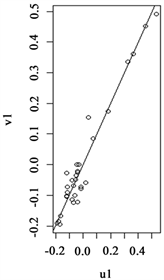Figure 6. Scatter diagram of variables

${U}_{1}=0.14345426{X}_{1}+0.02992152{X}_{2}+0.04527655{X}_{3}+0.02074174{X}_{4}$

$\begin{array}{c}{V}_{1}=0.027959833{Y}_{1}+0.002816796{Y}_{2}+0.124785324{Y}_{3}-0.016926069{Y}_{4}\\ +0.040482011{Y}_{5}-0.021746062{Y}_{6}+0.011092050{Y}_{7}+0.019115109{Y}_{8}\end{array}$

5.2. 结论部分

6. 结论及建议

Relevant Analysis on the Structure ofConsumption Expenditure in China’s Regions, Urban and Rural Areas[J]. 统计学与应用, 2018, 07(05): 521-532. https://doi.org/10.12677/SA.2018.75061

1. 1. 秦海林. 农村消费结构的户间差异与农户收入分配[J]. 财经科学, 2006(11): 90-96.

2. 2. 何平, 谢介仁. 中国家庭收入-消费关系研究[J]. 经济学动态, 2009(7): 32-35.

3. 3. 彭海艳. 影响中国农村居民消费结构的多因素实证分析[J]. 财贸研究, 2009, 20(1): 30-35.

4. 4. 李鹏, 曾光. 我国农村居民消费结构变动的实证分析[J]. 华中农业大学学报(社会科学版), 2011(6): 50-54.